An Aggregated result calculation is available in the StoryTeller charts and tables. The feature is like the existing Mean value series function, but it sums the values displayed in the chart/table instead of calculating the mean value. The feature can be used to for example aggregate the result of questions to create an index or aggregate answers to create a top two box.

As the feature works in same way as Mean value series you can select if the Aggregates series will be shown in columns or rows (in tables) and in axes or legend (in charts). You can also select if the original values, the values that have been aggregated, shall be displayed or removed. Below you see a few different use cases where the new Aggregated series function have been used.

In this example the new Aggregated series function has been used to create and calculate the Top box value (usually a Top box is created via a Grouped answer but now you can use the aggregated series function to do the same).

NB See note about rounding further down.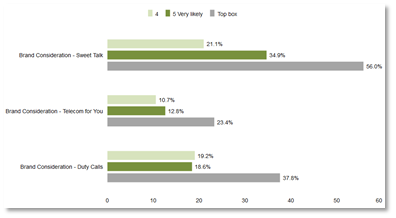In this example a Total score index has been created by using the Aggregated series function. The Total score is the sum of the three image attributes in this example. Refer also to rounding explanation below.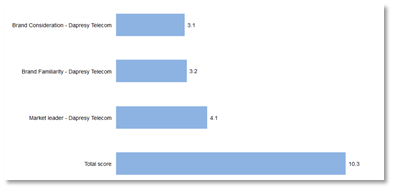The example below is the same as the one above but in this case the ingoing image variables have been hidden and a Solid gauge is used  to visualize the Total score result.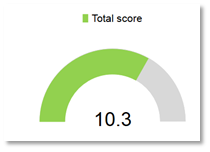The Aggregated series can be used in StoryTeller charts and tables and the setup is made in the analysis tab in same manner as when setting up Mean values series.

The interface for setting up the Aggregated series: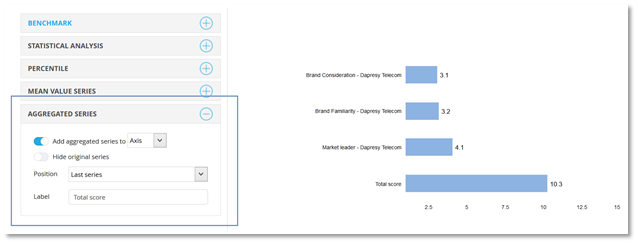To setup an Aggregated series follow the steps below:

• Select if the aggregation is to be on axis or legend (or rows or columns if it is a table object)

• Select if the original values shall be hidden or not

• If the Original series are not hidden you have to select position of the aggregated series, it can be displayed as the first or last series.

• Enter the label of the new aggregated series  (max 100 characters)

• If the Aggregated series is displayed in legend in a chart you also need to select the color of the new series.

The Aggregated series is function is summing up the values in the chart/table. The calculation uses all the decimals and not the rounded values displayed in the chart/table. In the image below you see an example of the calculation, as shown it uses all the decimals and not the rounded values.

Here we see an example of the calculation logic, all the decimals are used and not the rounded values.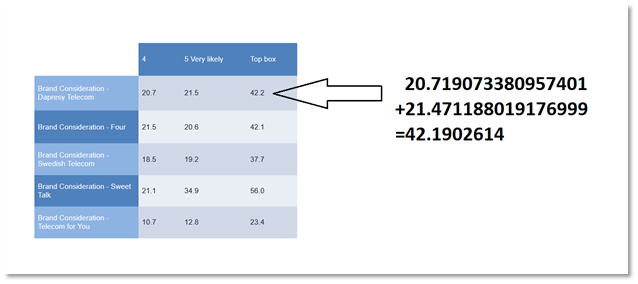Note: The aggregation is not performed if any of the in-going results are “missing” which can be the case when for example aggregating items with different base sizes. If the result is 0 the aggregation will be made. Below you find two examples of these use cases.

In this example the Aggregated series has been used to calculate the Total index based on the Sales and Service touchpoint indexes. As shown the Total index is not being calculated for Dealer C as Dealer C does not have any data for the Sales index.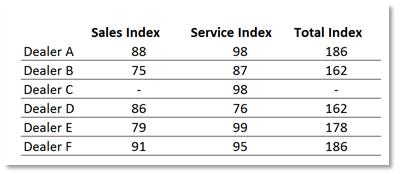In this example the Aggregated series has been used to calculate the Top box based on the 4 and 5. As shown the Top box is being calculated for Dealer C as the result of “4” is 0 and not missing.Note: in case Dapresy cannot aggregate data with system missings only. The workaround is to make sure the result will be 0 for those questions.